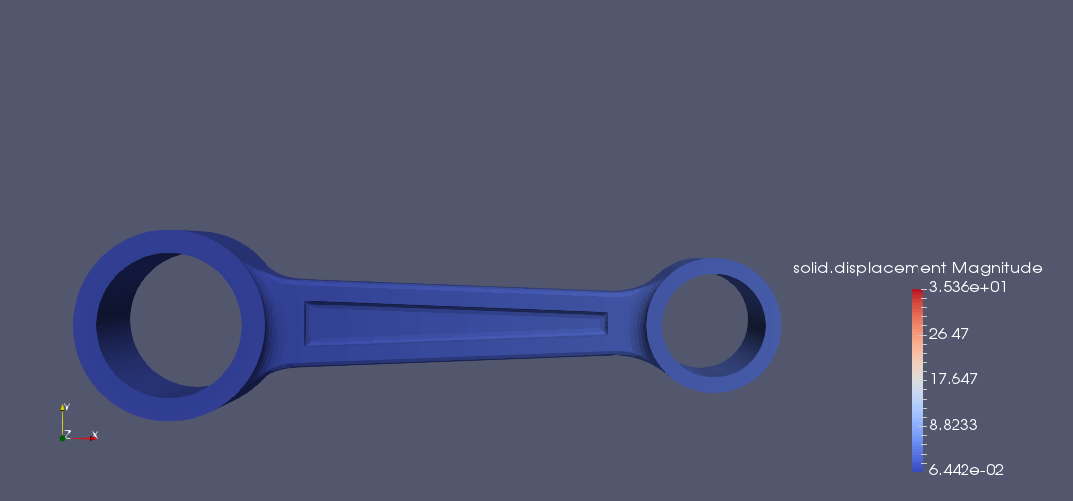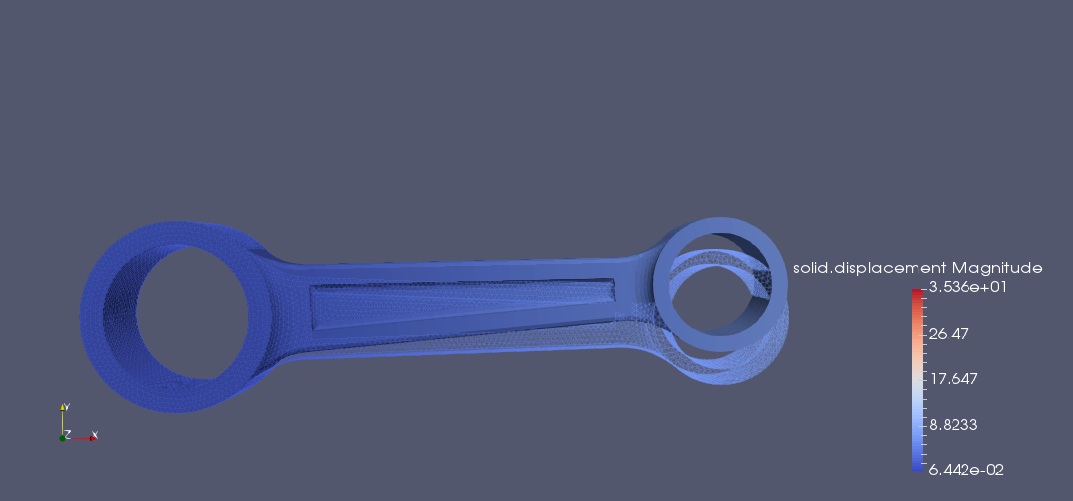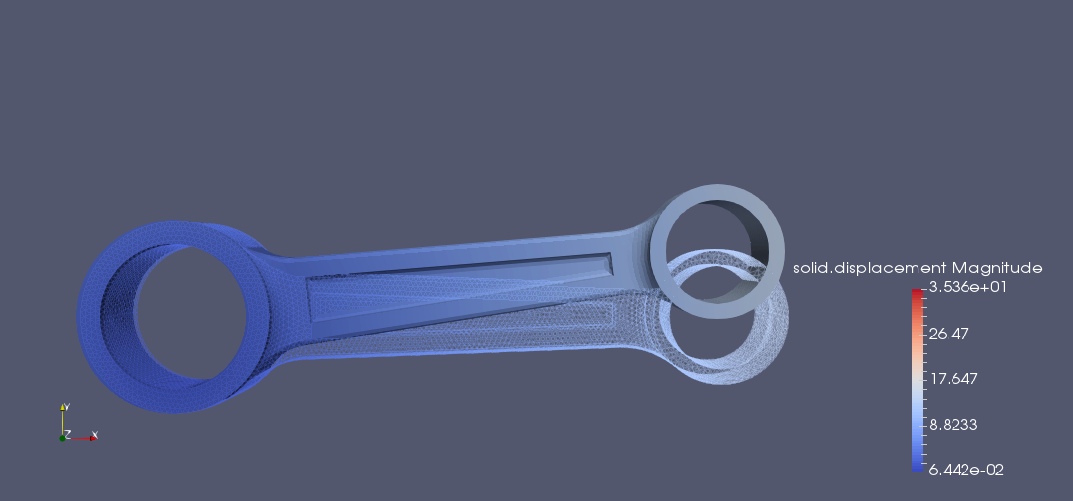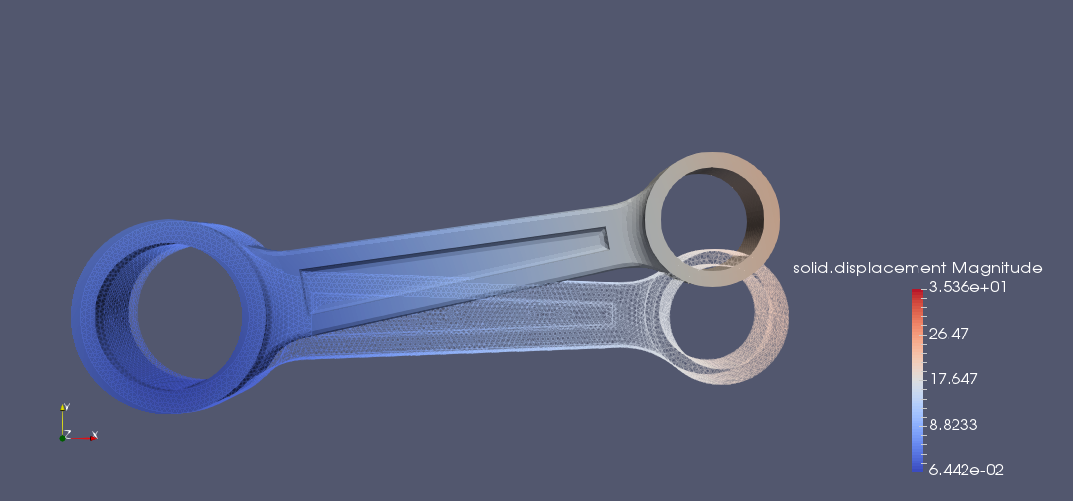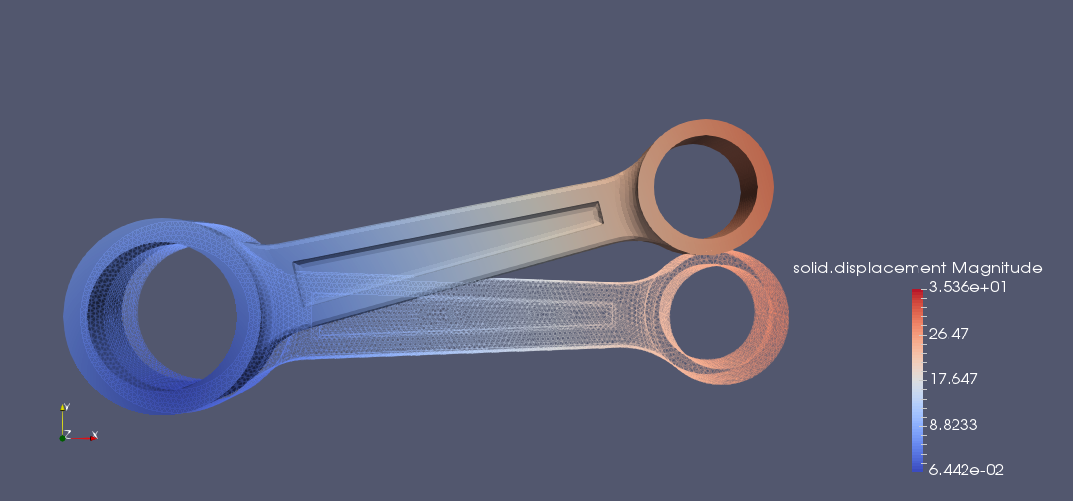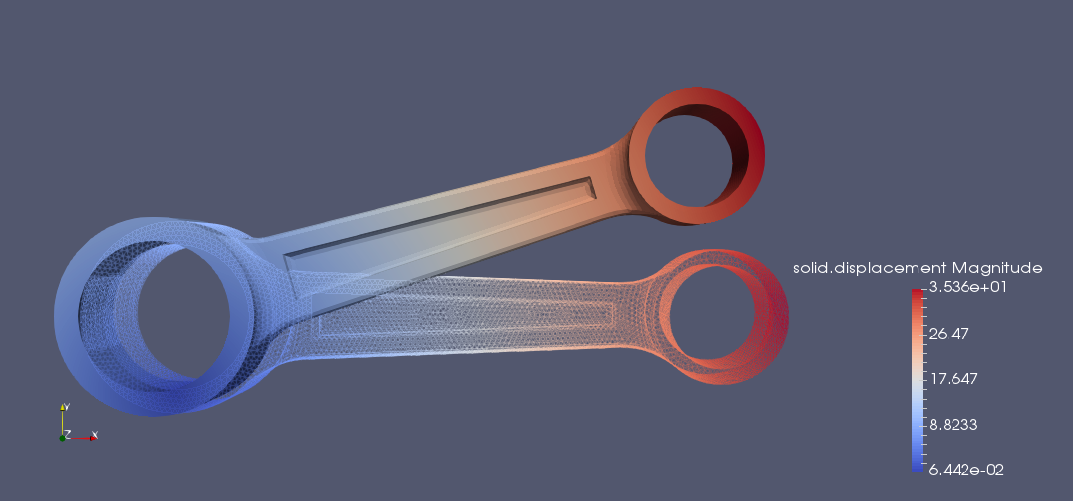# Rotating winch

## 1. Introduction

Simulate the rotation around the center of one of the holes in a winch.

(18 degree ~ 18°/180°*π = 0.31415 → 0.3)

## 2. Running the case

The command line to run this case is

``mpirun -np 4 feelpp_toolbox_solid --case "github:{repo:toolbox,path:examples/modules/csm/examples/rotating-winch}"``
Case option
`--case "github:{repo:toolbox,path:examples/modules/csm/examples/rotating-winch}"`

## 3. Data files

The case data files are available in Github here

## 4. Model/Geometry

The first step is to create the model of the object, which we can simply do in the Inventor program. With this program was the fastest and easiest way to create the model.

The finished geometry (Inventor) and the meshed model (Gmsh):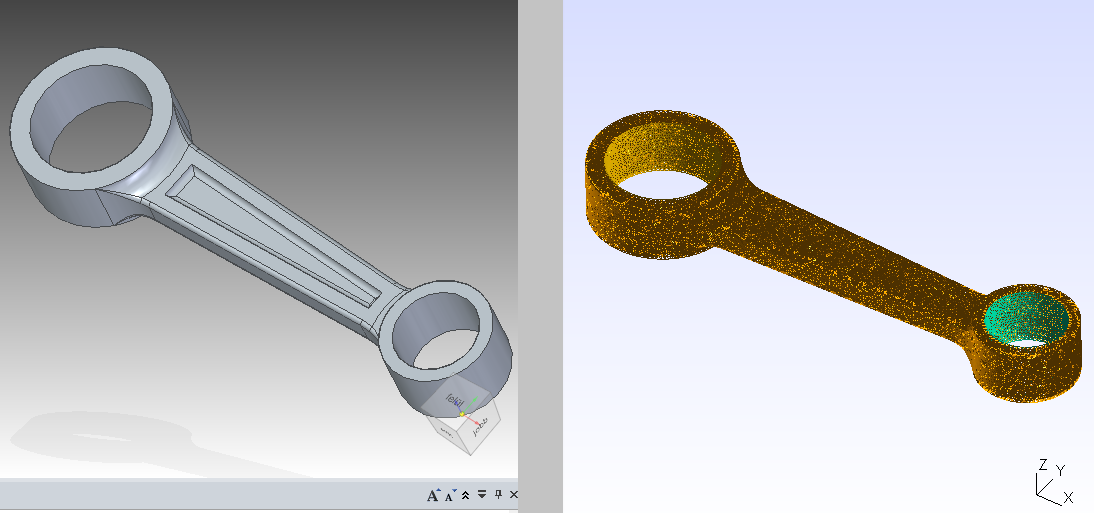## 5. Materials and boundary conditions

### 5.1. Materials

The beam is made of copper, so we need to use the following data:

• E = 124 GPa → 124e3 N/mm2

• nu = 0.33

• rho = 8920 kg/m3 → 8920e-9 kg/mm^3 ^

``````    "Materials":
{
"copper":{
"markers":"omega",
"E":"124e3",
"nu":"0.33",
"rho":"8920e-9"
}
},``````

### 5.2. Parameters

``````    "Parameters":
{
"rotation":
{
"value":"0.3" 	//pi/10=18 degree
},
"A":
{
"value":"-3.8"
},
"B":
{
"value":"0.17"
}
},``````

### 5.3. Boundary conditions

The center of one of the holes in a winch is fixed.

``````    "BoundaryConditions":
{
"displacement_x":
{
"Dirichlet":
{
{
"expr":"A + (x - A)*cos(rotation) - (y - B)*sin(rotation) - x :x:y:A:B:rotation"
}
}
},
"displacement_y":
{
"Dirichlet":
{
{
"expr":"B + (x - A)*sin(rotation) + (y - B)*cos(rotation) - y :x:y:A:B:rotation"
}
}
},
"displacement_z":
{
"Dirichlet":
{
{
"expr":"0"
},
"fixed":
{
"expr":"0"
}
}
}
},``````

## 6. Results

 The result were run in time (a whole period, but on the pictures can be seen only a half period).

### 6.1. Displacement